# Springs Notes | Study Mechanical Engineering SSC JE (Technical) - Mechanical Engineering

## Document Description: Springs for Mechanical Engineering 2022 is part of Strength of Materials (SOM) for Mechanical Engineering SSC JE (Technical) preparation. The notes and questions for Springs have been prepared according to the Mechanical Engineering exam syllabus. Information about Springs covers topics like and Springs Example, for Mechanical Engineering 2022 Exam. Find important definitions, questions, notes, meanings, examples, exercises and tests below for Springs.

Introduction of Springs in English is available as part of our Mechanical Engineering SSC JE (Technical) for Mechanical Engineering & Springs in Hindi for Mechanical Engineering SSC JE (Technical) course. Download more important topics related with Strength of Materials (SOM), notes, lectures and mock test series for Mechanical Engineering Exam by signing up for free. Mechanical Engineering: Springs Notes | Study Mechanical Engineering SSC JE (Technical) - Mechanical Engineering
 1 Crore+ students have signed up on EduRev. Have you?

SPRINGS
• Spring is a device, in which the material is arranged in such a way that it can undergo a considerable change, without getting permanently distorted.
• A spring is used to absorb energy due to resilience which may be restored as and when required.
• The quality of a spring is judged form the energy it can absorb. The spring which is capable of absorbing the greatest amount of energy for the given stress is the best one.
Stiffness of a Spring
• The load required to produce a unit deflection in spring is called spring stiffness.
Types of Springs
There are two types depending upon the type of resilience:
• Bending spring (leaf spring)
• Torsion spring (helical spring)
Leaf Springs
Central deflection dis given by,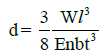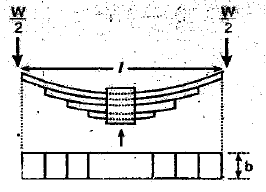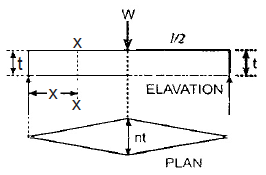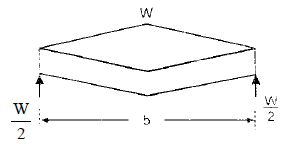Where,
l = Span of spring
t = thickness of plates
b = Width of plates
n = Number of plates
W = Load acting on the spring
E = Young's modulus
• Deflection in the spring due to load W,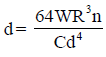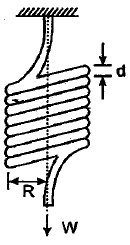• Stiffness of the spring,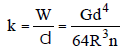Where,
n = no. of turns of spring
d = diameter of the rod of the spring
R = mean radius of the coil
G = modulus of rigidity for the spring material.
Energy stored,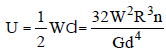Closely coiled helical spring subjected to axial twist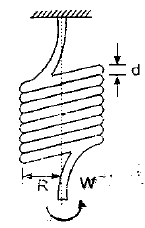• Total angle of bend =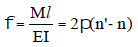where l = 2pRn = 2pR 'n '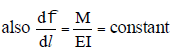constant
Thus, the change in curvature or angle of bend per unit length, is constant, throughout the spring.
• Energy stored =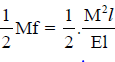Where,
R = mean radius of the spring coil
n = no. of turns or coils
M = Moment or axial twist applied on the spring
R = decreased mean radius due to twist
n = increased no of turns due to twist
I = moment of inertia of spring rod section
E = Young's modulus
Open coiled helical spring subjected to axial load
• In this case load W will cause both twisting and bending of coils.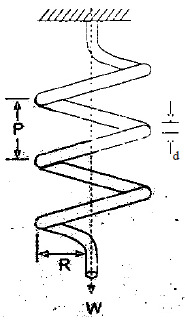• Deflection of the spring as a result as a result of axial load,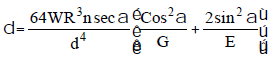Where,
d = Diameter of spring wire
R = Mean radius of spring coil
P = Pitch of the spring coil
n = no. of turns of coils
G = modulus of rigidity for spring material v
W = axial load on the spring
a = angle of helix
E = Young's modulus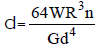= deflection of closed coiled spring.
SPRINGS IN SERIES AND PARALLEL
(i) Springs in series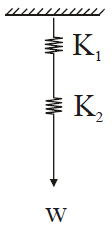• Total extension = å (individual extensions)
d= d1 + d2
• load applied (W) will be same on both the springs.
• The equivalent stiffness is given by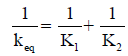where K1 & K2 are individual stiffness of the springs.
(ii) Springs in parallel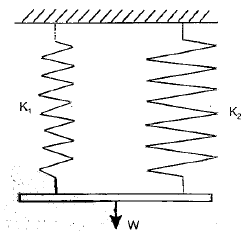• Both the springs have same extension.
d = d1 = d2
• Load applied (W) is shared by the springs.
W = W1 + W2
• The equivalent stiffness is given by,
eq.  K = K1 + K2

The document Springs Notes | Study Mechanical Engineering SSC JE (Technical) - Mechanical Engineering is a part of the Mechanical Engineering Course Mechanical Engineering SSC JE (Technical).
All you need of Mechanical Engineering at this link: Mechanical Engineering

## Mechanical Engineering SSC JE (Technical)

6 videos|89 docs|55 tests
 Use Code STAYHOME200 and get INR 200 additional OFF

## Mechanical Engineering SSC JE (Technical)

6 videos|89 docs|55 tests

Track your progress, build streaks, highlight & save important lessons and more!

,

,

,

,

,

,

,

,

,

,

,

,

,

,

,

,

,

,

,

,

,

;